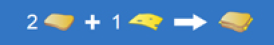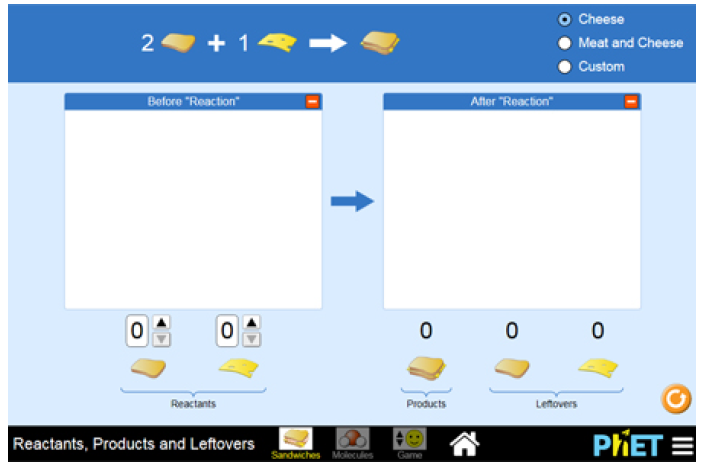Problem: In the Sandwiches mode, select the "Cheese" option and observe the equation given for the preparation of a cheese sandwich. In the equation, set the number of bread slices to "2" and the number of cheese slices to "1." You will see that the product formed by these three ingredients is one cheese sandwich.Suppose you have 42 bread slices and 32 cheese slices. How many cheese sandwiches can you make?In this simulation, select the Sandwiches icon where you can set the number of bread slices, cheese slices, and meat slices to prepare a sandwich. You can then move to the Molecules version where you will be able to relate the analogy to one of three specific chemical reactions.

FREE Expert Solution

For this problem, we are being asked to determine how many cheese sandwiches can you make using the given amount of bread and cheese

Recall that:79% (397 ratings)
Problem Details

In the Sandwiches mode, select the "Cheese" option and observe the equation given for the preparation of a cheese sandwich. In the equation, set the number of bread slices to "2" and the number of cheese slices to "1." You will see that the product formed by these three ingredients is one cheese sandwich.

Suppose you have 42 bread slices and 32 cheese slices. How many cheese sandwiches can you make?In this simulation, select the Sandwiches icon where you can set the number of bread slices, cheese slices, and meat slices to prepare a sandwich. You can then move to the Molecules version where you will be able to relate the analogy to one of three specific chemical reactions.